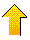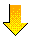1.  DAM BREACH FLOOD WAVE PROPAGATION• Vw = reservoir volume at the time of the breach, per unit of downstream channel width (Vw = 27000, 54000, 108000 m3[/ m]).

• So = downstream channel bed slope (So = 0.01, 0.001, 0.0001).

• qr = reference unit-width discharge (qr = 5 or 10 m2s-1, depending on Vw ).REFERENCE:

Ponce, V. M., A. Taher-shamsi, and A. V. Shetty. 2003. Dam-breach flood wave propagation using dimensionless parameters. ASCE Journal of Hydraulic Engineering, Vol. 129, No. 10, October, 777-782.

 2.  DIMENSIONLESS PARAMETERS• qp = peak discharge, per unit of channel width, immediately below the damsite (initial values at the damsite are qp = 5, 10, 20 o 40 m2s-1, depending on Vw ).

• qp/qr = ratio of peak discharge to reference discharge (initial values of 1, 2, and 4).

• Lo = reference channel length, i.e., the ratio of reference flow depth do to channel bed slope So (Lo = do / So ).

• X /Lo = distance or length, along downstream channel, normalized with respect to the reference channel length Lo.3.  SAMPLE CALCULATION• Given: Vw = 54,000 m3/ m; So = 0.001.

• Figure 2 (b) shows the relations qp/qr vs X /Lo for various slopes and qr = 10 m2s-1.

• The ultimate normalized peak discharge  (qp/qr )u = 0.375 corresponds to the ultimate normalized distance (X /Lo ) u = 20.

• The ultimate peak discharge  (qp )u = 0.375 × qr = 0.375 × 10 = 3.75 m2s-1.4.  SAMPLE CALCULATION (continued)• The unit-width discharge (Manning equation) is:   q =  (1/n ) do5/3 So1/2

• The ultimate reference flow depth is:   (do )u = (qu n  / So1/2 )3/5

• A value of Manning n = 0.05 is assumed (applicable to natural conditions in middle-slope valleys).

• The ultimate reference flow depth is:   (do )u =   (3.75 × 0.05 / 0.0011/2)3/5 =   2.91 m.5.  SAMPLE CALCULATION (continued)• The reference channel length, based on the ultimate discharge, is:
(Lo )u = (do )u / So =   2.91 / 0.001 =   2,910 m.

• The ultimate distance or length is: Xu =  (X /Lo)u (Lo )u = 20 × 2,910 = 58,200 m ≅ 58.2 km.6.  PRACTICAL EXAMPLE• The failure of Teton Dam, in June 5, 1976, produced a peak discharge at the damsite of 2,300,000 cfs.

• About 100 miles downstream, the peak discharge had attenuated to 50,000 cfs, or about 2% of the original value.Failure of Teton Dam, on the Teton River, Idaho, June 5, 1976.

REFERENCE:

Ponce, V. M., A. Taher-shamsi, and A. V. Shetty. 2003. Dam-breach flood wave propagation using dimensionless parameters. ASCE Journal of Hydraulic Engineering, Vol. 129, No. 10, October, 777-782.

 090208